## Example Questions

### Example Question #1 : How To Find The Diameter Of A Sphere

A cube with a surface area of 216 square units has a side length that is equal to the diameter of a certain sphere. What is the surface area of the sphere?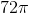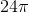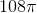Explanation:

Begin by solving for the length of one side of the cube. Use the formula for surface area to do this: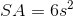s= length of one side of the cube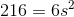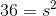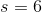The length of the side of the cube is equal to the diameter of the sphere. Therefore, the radius of the sphere is 3. Now use the formula for the surface area of a sphere: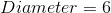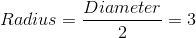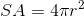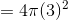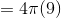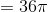The surface area of the sphere is.

### Example Question #2 : How To Find The Diameter Of A Sphere

The surface area of a sphere is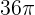. What is its diameter?Explanation:

The surface area of a sphere is defined by the equation:For our data, this means: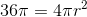Solving for, we get: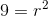or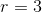The diameter of the sphere is.

### Example Question #3 : How To Find The Diameter Of A Sphere

The volume of one sphere is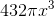. What is the diameter of a sphere of half that volume?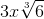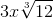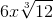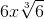Explanation:

Do not assume that the diameter will be half of the diameter of a sphere with volume of. Instead, begin with the sphere with a volume of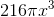Such a simple action will prevent a vexing error!

Thus, we know from our equation for the volume of a sphere that: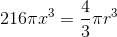Solving for, we get: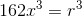If you take the cube-root of both sides, you have: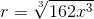First, you can factor out an: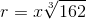Next, factor the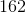: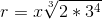Which simplifies to: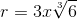Thus, the diameter is double that or:Tired of practice problems?

Try live online GRE prep today.